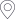﻿ 师资概况-华中师范大学物理科学与技术学院网站首页> 学院概况

站内搜索

 陈继胜(教授) 计晨(副教授) 罗晓峰(副教授) 吴青林(副教授) 余兰山(副教授) 陈绍龙(教授) 贾 亚(教授) 毛亚显(副教授） 吴少平（教授） 余颖(教授) 陈小宇（副教授） 金猛(副教授) 裴 骅(副教授) 吴彦文(教授) 俞云伟(教授) 陈绪君(副教授) 瞿少成(教授) 乔翠兰(副教授) 吴元芳(教授) 詹璇 程筠 李高翔(教授) 秦广友 夏应清(教授) 张本威(教授) 池丽平(副教授) 李玲远(教授) 邱明(副教授) 谢跃红(教授) 张冬亮（副教授） 邓为炳(副教授) 李炜(教授) 邱涛涛(副教授) 徐洪波(副教授) 张国平(教授) 丁亨通(教授) 李新强(教授) 施梳苏(教授) 徐俊(副教授) 张汉中(教授) 段传喜(教授) 李勇(教授) 石丽华(副教授) 许明梅(副教授) 张青林 冯秀梅(副教授) 李志扬(教授) 孙向明(教授) 薛钦(副教授) 张晓明 付菁华(教授) 李治明(副教授) 谭华堂(教授) 殷中宝(教授) 张新晨(副教授) 郭迪(副教授) 林海(副教授) 谭明新(副教授） 杨纯斌(教授) 赵蕴杰(副教授) 郭红(教授) 凌毓涛(副教授) 谭 铭(教授) 杨河林(教授) 郑小平(教授) 侯德富(教授) 刘峰(教授) 唐一文(教授) 杨利建（副教授） 周爱芝 侯喜文(教授) 刘复明 王东 杨林涛 周代翠(教授） 胡君红副教授 刘复明(教授) 王恩科 杨苹(副教授) 周代梅(教授) 胡响明(教授) 刘 蓉(副教授) 王建中(副教授) 杨书华 祝志宏(副教授) 黄光明(教授) 刘守印(教授） 王新年 杨亚东(教授) 黄新堂(教授) 刘延申(教授) 王亚平 （教授） 姚 远(副教授) 黄致新(教授) 罗小巧(副教授) 吴建斌(副教授) 尹航(教授)